# A New Adaptive Neuro-Fuzzy Inference System (ANFIS) and PI Controller to Voltage Regulation of Power System Equipped by Wind Turbine

A New Adaptive Neuro-Fuzzy Inference System (ANFIS) and PI Controller to Voltage Regulation of Power System Equipped by Wind Turbine

Issam GricheSabir Messalti Kamel Saoudi Mohamed Y. Touafek

Department of Electrical Engineering, Faculty of technology, University of M’sila, M’sila, Algeria

Department of Electrical Engineering, Faculty of Engineering, University of Bouira, Bouira, Algeria

Corresponding Author Email:
issam.griche@univ-msila.dz
Page:
149-155
|
DOI:
https://doi.org/10.18280/ejee.210204
26 January 2019
|
Accepted:
29 March 2019
|
Published:
30 June 2019
| Citation

OPEN ACCESS

Abstract:

In this paper, new Adaptive Neuro-Fuzzy Inference System (ANFIS) and PI controller have been proposed and investigated which the power system is equipped by Static Var Compensator (SVC) and small wind turbine. The SVC is controlled by PI controller optimized by genetic algorithm (GA) to regulate the voltage profile. To demonstrate the efficiency of proposed controller, a Single Machine Infinite Bus (SMIB) has been considered, in which small fluctuation of mechanical damped has been applied to improve the transient stability and has been evaluated using a relative rotor criteria. Obtained results have demonstrated a better performance with ANFIS and PI controller in which both voltage and transient stability have been controlled perfectly.

Keywords:

power network, distributed generator (DG), simulation, intelligent controller

1. Introduction

In the last few years, Electro-energetic systems are often subject to disturbances that can cause serious problem; their reliability is defined by operating limits and behavior subject disturbances [1-3]. These disturbances lead to additional constraints, mechanical, thermal, or electrical to those of the steady state and give the instability of the power system. Quite recently, considerable attention has been paid to cope with its problems, two means of improving stability, classical means such us transformer with taps adjustable, shifting phase transformer and modern means such us using a controller in the generator which an additional signal control in the exciter system of the generator, or using a controller in line-side which additional control signal in the systems FACTS devices. Due to previous dilemma, the power systems require more and more advanced devices and techniques. Current research on power system improvement is focused on different techniques such as HVDC, FACTS, and PSS [4-7].

Several publications have appeared in recent years documenting the PSSs. The literature on  shows a variety of approaches such as PID and lead lag compensators  which have been devoted to the study of optimization algorithm. One of the first examples of PSO is presented in . The results obtained in [10-15] suggest that the sequential linear programing such as Genetic Algorithm (GA), chaotic algorithm, bacteria foraging and H∞. A key limitation of this research is that their simple structure, need to an accurate model and its non-optimal damping in different cases of power system.

However, the authors are available in the literature that discussed the issue of the adaptive of the PSS such as, neural networks (NN) [16-19], Wavelet neural networks (WNN) controller [20-21], self-tuning PID controllers [22-23], sliding mode and fuzzy controller [24-25]. Nevertheless, there are still some interesting and relevant problems to be addressed using adaptive control law. The problem with this approach is in that it requires offline training. Hence, an appropriate PSS have indicated in view of the drawbacks.

Among them, Flexible alternating current transmission systems (FACTS) devices have proved to be very effective and viable in alleviating the problems of electrical transmission systems for voltage controller and transient stability [26-27], security, and reliability in the modern power systems [28-29]. In addition, Distributed Energy Systems  has on the centralized supply in terms of cost of the energy delivered to consumers is evaluated.

To solve these defects, this paper establishes a new Adaptive Neuro-Fuzzy Inference System (ANFIS) and PI controller to improve the transient stability and regulate the voltage profile of SMIB connected to small wind turbine and SVC. The propped ANFIS Controller is used to control the excitation system of generator; the SVC is controlled by PI optimize by genetic algorithm for load bus voltage improvement. Furthermore, assessment of power system transient stability and voltage regulation is studied in case of small fluctuation of mechanical power.

The remainder of this paper is organized as follows: Section 2 introduces the model of power system under studied. Section 3, describes the conventional PSS structure (CPSS). Section 4 provides the design of proposed controllers. Section 5 summarizes the results of this work and draws conclusions.

2. Power System Modeling Under Study

The efficiency of the proposed method has been tested using Single Machine infinite Bus (SMIB) equipped by SVC and a small wind turbine is connected in bus 2 shown in Figure 1. The external line parameters, $R_{e}$ and $X_{e}$ are to be varied to change the electrical strength of the connection between the synchronous generator and infinite bus which the load, wind turbine and the SVC are connected.

## 1.jpgFigure 1. SMIB equipped by SVC and wind turbine

To demonstrate the validity of the proposed method, the standard two-axis model of the generator with field winding on the direct axis and damper winding on the quadrature axis, an IEEE type-I excitation system and a simplified turbine/governor model are adopted in this study. 

The stator voltage equations with the external impedance included is:

\begin{align} & {{v}_{q}}=-\left( {{r}_{s}}+{{R}_{e}} \right){{I}_{q}}-\left( x_{d}^{'}+{{X}_{e}} \right){{I}_{d}}+E_{q}^{'} \\ & {{v}_{d}}=-\left( {{r}_{s}}+{{R}_{e}} \right){{I}_{d}}-\left( x_{q}^{'}+{{X}_{e}} \right){{I}_{q}}+E_{d}^{'}\, \\ \end{align}       (1)

$\dot{\delta }=\left( \omega -{{\omega }_{b}} \right)$         (2)

$\dot{\omega }=\frac{{{\omega }_{b}}}{2H}\left[ {{P}_{m}}-{{P}_{e}}-D\,\left( \omega -{{\omega }_{b}} \right) \right]$           (3)

${{\dot{{E}'}}_{q}}=\frac{1}{T{{'}_{d0}}}\left[ {{V}_{ex\,}}-E{{'}_{q}}+\left( {{x}_{d}}-x{{'}_{d}} \right)I{{'}_{d}} \right]$        (4)

${{\dot{{E}'}}_{d}}=\frac{1}{T{{'}_{q0}}}\left[ -E{{'}_{q}}-\left( {{x}_{q}}-x{{'}_{q}} \right)I{{'}_{q}} \right]$             (5)

The electrical power at the bus 2 is given by:

${{P}_{e}}={{P}_{gen}}+{{P}_{wind}}$         (6)

where: $P_{g e n}$ is the electrical power generated by the synchronous generator, $P_{\text {wind}}$ is the electrical power generated by the wind turbine which the captured power (in W) by a Wind turbine (WT) can be written by:

${{P}_{wind}}=\frac{1}{2}{{\rho }_{w}}.{{A}_{rw}}.V_{w}^{3}.{{C}_{pw}}({{\lambda }_{w}},{{\beta }_{w}})$        (7)

where $\rho_{w}$ the air density (kg/m3), $A_{r w}$ is the blade impact area (m2), $V_{w}$ is the wind speed (m/s), the WT $\lambda_{w}$ the tip speed ratio, $\beta_{w}$ is blade pitch angle (degrees) and $C_{p w}$ power coefficient.

where $r_{S}, H, D, \cdot \omega, \cdot \delta, P_{m}, \cdot P_{e}$ are the parameters of the machine given in .

For the regulation of the voltage at bus 2, the model is completed by the algebraic equation expressing the reactive power injected $Q_{S V C}$ at the SVC node: 

${{Q}_{SVC}}=-{{b}_{SVC}}V_{2}^{2}$         (8)

where $b_{S V C}$ is the susceptance,

The regulator has an anti-windup limiter. Thus, the reactance $b_{S V C}$ is locked if one of its limits is reached and the first derivative is set to zero shown in Figure 2.

Let us define the following dependence of shunt-connected capacitor raises the voltage. The value of $b_{S V C}$ or $\mathcal{Y}_{S V C}$ is obtained by PI controller (Figure 2).

## 2.jpg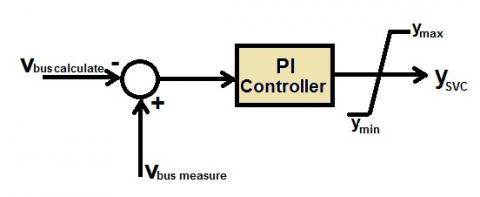Figure 2. SVC with PI controller

To verify the validity of the PI controller method, we carried out the power system shown in Figure 1, voltage source of synchronous generator $V_{1}$ and an impedance of the transmission line $R_{e}+j X_{e}$. The equation that describes the voltage compensating impedance at the bus 2 $V_{2}$ is as follows:

${{V}_{2}}=\frac{1}{{{y}_{22}}+{{y}_{SVC}}}\left[ \frac{\left( P-{{P}_{wind}} \right)-j\left( Q\pm {{Q}_{SVC}} \right)}{{{V}_{2}}}-{{y}_{12}}{{V}_{1}} \right]$  (9)

where $y_{S V C}, y_{22}, y_{12}$ are the admittance of the SVC, bus 2 and the transmission line.

To resolve and calculate and the bus voltage $V_{2}$ Gauss-Sidel method have been used.

Therefore, there is a need for an effective method for tuning the parameters of the PI controllers so as to maximize the voltage regulation of power system. Genetic Algorithm (GA) is a global searching technique based on the operations observed in natural selection and genetics. The stage of GA application is PI controller set parameters tuning, since each PI controller has one input. The chromosome length in this stage will be (27). Table (I) shows GA parameters in scaling factors tuning stage.

Table 1. GA parameters in scaling factors tuning stage

 Variable number 9 Gene number for each variable 3 Chromosome length 27 Population size 54 Generation number 50

The proposed algorithm flowchart is shown in Figure 3.

## 3.jpg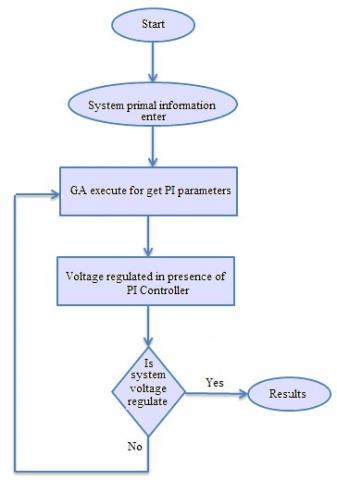Figure 3. Proposed algorithm flowchart

3. Conventional PSS Design (CPSS)

The bloc of CPSS consists of a stabilizer gain $K_{P S S}$, a washout block $\left(T_{W}\right)$ serves as a low-pass filter with a time constant and the phase compensation blocks with time constants $\left(T_{1}, T_{4}\right)$. From the Figure 4, the input signal of each PSS is the rotor speed deviation $(\Delta \omega(s y n))$ and the output is the stabilizing signal $\left(V_{s u p}\right)$ subject to an anti-windup limiter $V_{\sup }^{\min },V_{\sup }^{\max }$.

${{V}_{\sup }}=\left[ {{K}_{PSS}}\left( \frac{s{{T}_{w}}}{1+s{{T}_{w}}} \right)\left( \frac{1+s{{T}_{1}}}{1+s{{T}_{2}}} \right)\left( \frac{1+s{{T}_{3}}}{1+s{{T}_{4}}} \right) \right]\Delta \omega (syn)$ (10)

## 4.jpg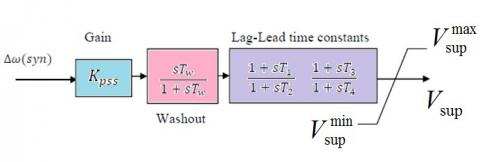Figure 4. Control structure of PSS

4. Design of Adaptive Neuro-Fuzzy Inference System (Anfis) Proposed

To achieve the best dynamical response of power system, the structure of excitation system of generator is displayed in Figure 5. The ANFIS controller can be used to add damping to the rotor oscillations of the synchronous generator by controlling its excitation. The ANFIS input signal can be the rotor speed deviation of generator ω.

To ensure a robust damping; the ANFIS controller should provide a moderate phase advance at frequencies of interest in order to compensate for the inherent lag between the field excitation and the electrical torque induced by the ANFIS action.

## 5.jpg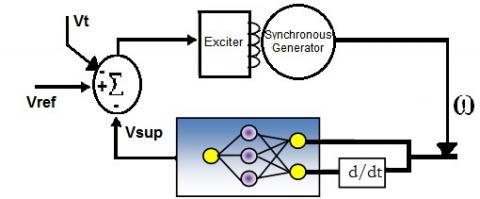Figure 5. Exciter system

The excitation system of the synchronous machine is represented by the following transfer function:

$\frac{{{V}_{ex}}}{{{E}_{f}}}=\frac{1}{{{K}_{E}}+s{{T}_{E}}}$       (11)

where $K_{E}$ and $T_{E}$ are respectively constant gain and time constant of exciter.

In standard excitation systems, to achieve the desirable dynamic performance and to shape the regulator response, a stabilizing circuit is used, which characterized by a gain $K_{F}$ and with a time constant $T_{F}$.

## 6.jpg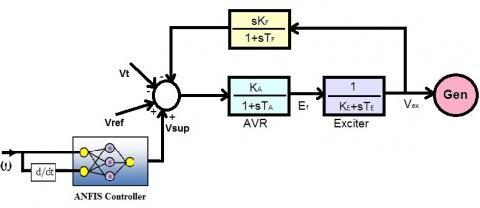Figure 6. Transfer function of excitation system

## 7.jpg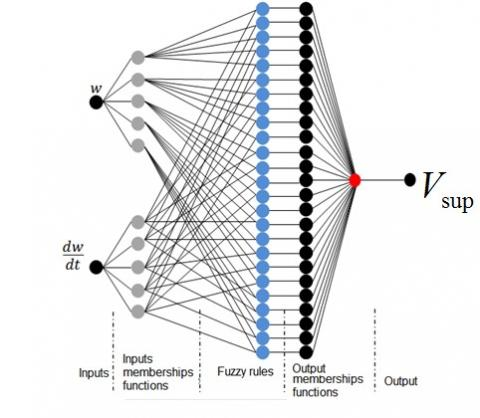Figure 7. Proposed ANFIS controller

## 8.jpg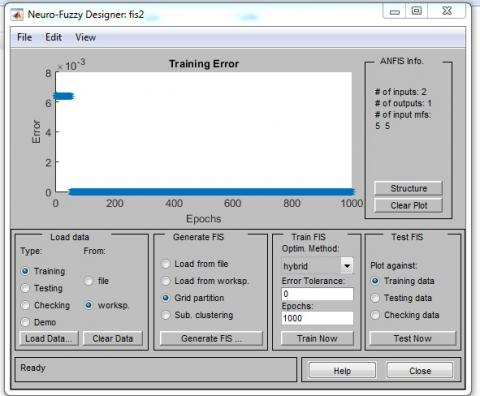Figure 8. Proposed ANFIS controller

The proposed strategy of control is composed ANFIS a controller, the inputs of this controller are oscillation of ω and its error. Figure 7 shows normalized membership functions for input and output variables.

Adaptive Neuro-Fuzzy Inference System (ANFIS) is a combining between Neural Networks and Fuzzy Inference System (FIS), it can be applied to solve several optimization problems.

In this paper, the $V_{S u p}$ is optimized by the Neuro-Fuzzy Controller shown in Figure 7. The training data which the speed of synchronous generator N1 is transmitted to the fuzzy logic toolbox using the conventional regulator shown in Figure 4. Then, using the ANFIS, the optimal fuzzy function is calculated using a hybrid method (back propagation error and least squares) according to the diagram shown in Figure 7. This provides, after learning process, the calculated functions of the two inputs and calculates the fuzzy rules that connect the inputs with the controller output. Since the fuzzy inference is Sugeno type, the output is a linear function with three coefficients:

If input 1 is Z and input 2 is P then output is $(a \times Z+b \times P+c)$ (a, b, c) are coefficients calculated by learning.

The fuzzy controller in our case contains 25 rules used to link inputs to the outputs. The Artificial neural networks (ANN) are used to adapt the membership functions and the determination of fuzzy rules. The configuration is Adaptive Neuro-Fuzzy Inference Systems (ANFIS). The method used for the Neural-fuzzy system adaptation is called hybrid: a combination of optimization by the least squares method and refined by the back propagation of the error.

The ANFIS Editor is used to create, train, and test a Sugeno fuzzy system shown in Figure 8.

The membership function obtained by ANFIS is shown in Figure 9.

## 9.jpg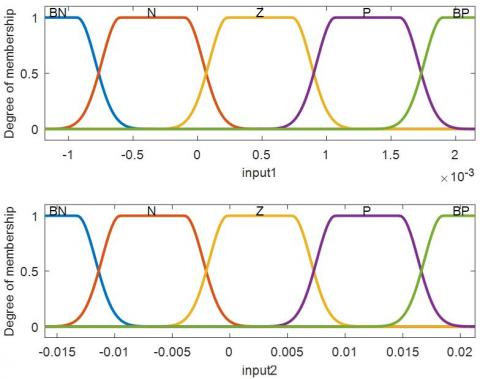Figure 9. Input Membership function Speed changes ω and its derivative

5. Simulation Results

In order to demonstrate the effectiveness of the proposed controller, the system being studied is simulated by MATLAB software shown in Figure 10.

## 10.jpg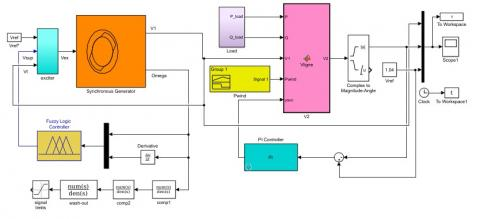Figure 10. System studied simulated by MATLAB software

The Flowchart for optimization of stochastic simulation is shown in Figure 11.

## 11.jpg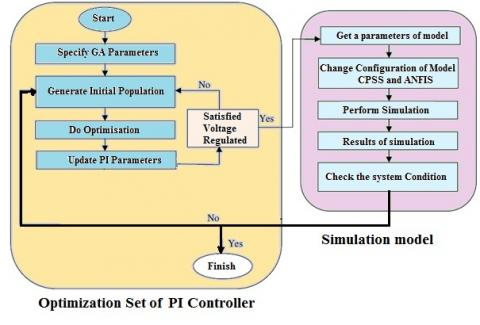Figure 11. Flowchart for optimization of stochastic simulation

SVC and wind turbine are connected to the bus load which the system has been designed to study the electromechanical oscillations in large interconnected power systems. It has also been modified to include FACTS devices for studying the damping voltage improvement which the SVC will maintain the load voltage profile to close to 1.04 p.u.

The simulation results show the principle of CCT calculation in which we increase and decrease the duration of the mechanical damping until the relative rotor angles become not oscillators weakened.

## 12a.jpg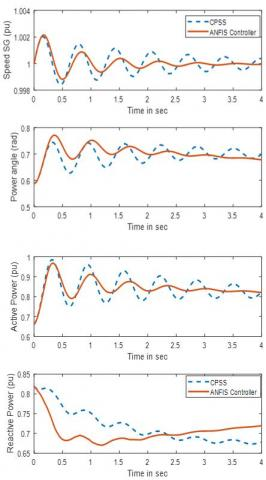Figure 12. Simulation results Tf=0.1, DT=5 %

The Figure 12 present the speed, the relative rotor angles, the active power and reactive power of synchronous machine in the case of fault duration Tf=0.1s and the size of the torque perturbation about operating point is 5 % of nominal torque.

In this section, we present the voltage improvement using SVC controlled by conventional PI optimize by GA, Figure 13 shown the voltage profile at the bus 1 and 2 for both cases: with and without of SVC device. It can be observed that the performance of voltage with SVC is better. So, the proposed strategy can be as one of the best methods for power system voltage control.

## 13.jpg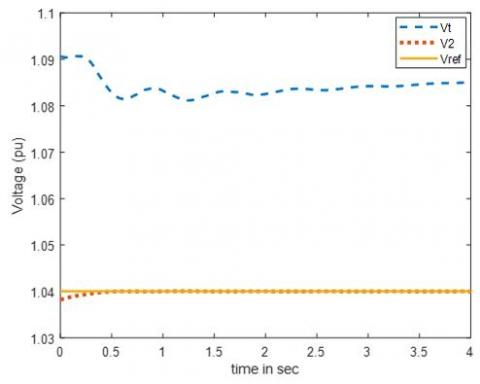Figure 13. Voltage controller

To regulate the voltage in power system, other simulation has been tested which the profile of our reference voltage has changed. In Figure 14, it can be noted that the performance of voltage regulation with SVC is better and the proposed PI controller improve the voltage regulation for power system.

## 14.jpgFigure 14. Voltage profile

6. Conclusions

In this paper, Adaptive Neuro-Fuzzy Inference System (ANFIS) and PI controller have been proposed and investigated which the power system is equipped by Static Var Compensator (SVC) and small wind turbine. The detailed architecture and the proposed method were discussed using SMIB which the SVC is controlled by PI controller optimize by Genetic Algorithm (GA). The simulation results demonstrate the efficiency of proposed controller in which the evaluation of the transient stability has been based on relative rotor angle criteria and the voltage regulation. The obtained results confirm the performance of the proposed ANFIS and PI controller. In future researches, this work can be completed using multi machine power system and optimization of PI controller using other technics.

References

 Ramírez-Arredondo, J.M. (2000). The closed loop characteristic polynomial as a means to obtain robust performance of conventional PSS. Electr Power Energy Syst, 22: 259-68. https://doi.org/10.1016/S0142-0615(99)00056-3

 Kundur, P. (1994). Power System Stability and Control. McGraw Hill, New York.

 Concordia, C, de-Mello, F.P. (1969). Concepts of synchronous machine stability as affected by excitation control. IEEE Trans Power Appar Syst; PAS-88, 316-329. https://doi.org/10.1109/TPAS.1969.292452

 Divya, A., Pallavi, C., Malvika, C. (2015). Performance evaluation and improvement in transient instability of IEEE 9 bus System using exciter and governor control. Procedia Computer Science, 70: 733-739. https://doi.org/10.1016/j.procs.2015.10.111

 Bo, Z.O., Aggarwal, K., Johns, A.T., Zhang, B.H., Ge, Y.Z. (1997). New concept in transmission line reclosure using high frequency fault transients. IET Proceedings - Generation Transmission and Distribution, 144(4): 351-356. https://doi.org/10.1049/ip-gtd:19971102

 Ioan, F., Octavian, P., Cristian, V., Iosif, S. (2016). An improved structure of an adaptive excitation control system operating under short-circuit. Advances in Electrical and Computer Engineering, 16(2): 43-50. https://doi.org/10.4316/AECE.2016.02006

 Balasingh, M.M., Ramachandran, V., Lakshmi, P. (2011). An effective distributed model for power system transient stability analysis. Advances in Electrical and Computer Engineering, 11(3): 71-76. https://doi.org/10.4316/AECE.2011.03012

 Hassan, B., Takashi, H., Hossein, B. (2011). Robust PID based power system stabiliser: Design and real-time implementation. International Journal of Electrical Power & Energy Systems, 33(2): 179-188. https://doi.org/10.1016/j.ijepes.2010.08.013

 Hossam, E., El-Sharkawy, M.A., Emary, A., Yassin, K. (2012). Design and allocation of power system stabilizers using the particle swarm optimization technique for an interconnected power system. International Journal of Electrical Power & Energy Systems, 34(1): 57-65. https://doi.org/10.1016/j.ijepes.2011.09.005

 Hassan, L.H., Moghavvemi, M., Almurib, H.A., Muttaqi, K.M., Ganapathy, V.G. (2014). Optimization of power system stabilizers using participation factor and genetic algorithm. International Journal of Electrical Power & Energy Systems, 55: 668-679. https://doi.org/10.1016/j.ijepes.2013.10.026

 Shayeghi, H., Shayanfar, H.A., Jalilzadeh, S., Safari, A. (2010). Multi-machine power system stabilizers design using chaotic optimization algorithm. Energy Conversion and Management, 51: 1572-1580. https://doi.org/10.1016/j.enconman.2010.02.015

 Mohammadi, M., Ghadimi, N. (2015). Optimal location and optimized parameters for robust power system stabilizer using honeybee mating optimization. Complexity, 21(1): 242-258. https://doi.org/10.1002/cplx.21560

 Mishra, S., Tripathy, M., Nanda, J. (2007). Multi-machine power system stabilizer design by rule based bacteria foraging. Elec Power Syst Res, 77(12): 1595-1607. https://doi.org/10.1016/j.epsr.2006.11.006

 Hardiansyah, F.S., Furuya, S., Irisawa, J. (2006). A robust H∞ power system stabilizer design using reduced-order models. Elect Power Energy Syst, 28(1): 21-28. https://doi.org/10.1016/j.ijepes.2005.09.002

 Jabr, R.A., Pal, B.C., Martins, N., Ferraz, J.C.R. (2010). Robust and coordinated tuning of power system stabiliser gains using sequential linear programming. IET Gener. Transm. Distrib, 4(8): 893–904. https://doi.org/10.1049/iet-gtd.2009.0669

 Alizadeh, M., Kojori, S.S., Ganjefar, S. (2013). A modular neural block to enhance power system stability. Power Systems, IEEE Transactions, 28(4): 4849-4856. https://doi.org/10.1109/TPWRS.2013.2278377

 Darabian, M., Jalilvand, A. (2017). A power control strategy to improve power system stability in the presence of wind farms using FACTS devices and predictive control. Electr Power Energy Syst, 85: 50-66. https://doi.org/10.1016/j.ijepes.2016.08.002

 HsuI, C.F., Lee, T.T., Tanaka, K. (2015). Intelligent nonsingular terminal sliding-mode control via perturbed fuzzy neural network. Engineering Applications of Artificial Intelligence, 45: 339–349. https://doi.org/10.1016/j.engappai.2015.07.014

 Saoudi, K., Harmas, M.N. (2014). Enhanced design of an indirect adaptive fuzzy sliding mode power system stabilizer for multi-machine power systems. International Journal of Electrical Power & Energy Systems, 54: 425-431. https://doi.org/10.1016/j.ijepes.2013.07.034

 Segal, R., Sharma, A., Kothari, M.L. (2004). A self-tuning power system stabilizer based on artificial neural network. Elect Power Energy Syst, 26(6): 423–30. https://doi.org/10.1016/j.ijepes.2003.11.010

 Liu, W.X., Venayagamoorthy, G.K., Wunsch II, D.C. (2003). Design of an adaptive neural network based power system stabilizer. Neural Networks, 16(5-6): 891-898. https://doi.org/10.1016/S0893-6080(03)00129-1

 Ganjefar, S., Alizadeh, M. (2012). A novel adaptive power system stabilizer design using the self-recurrent wavelet neural networks via adaptive learning rates. European Transactions on Electrical Power, 23(5): 601-619. https://doi.org/10.1002/etep.1616

 Farahani, M. (2013). Intelligent control of SVC using wavelet neural network to enhance transient stability. Engineering Applications of Artificial Intelligence, 26(1): 273-280. https://doi.org/10.1016/j.engappai.2012.05.006

 Farahani, M. (2013). A multi-objective power system stabilizer. IEEE Transactions on Power Systems, 28(3): 2700-2707. https://doi.org/10.1109/TPWRS.2012.2227980

 Hsu, Y.Y., Liou, K.L. (1987). Design of self-tuning PID power system stabilizers for synchronous generators. IEEE Trans Energy Conversion, EC-2(3): 343-348. https://doi.org/10.1109/MPER.1987.5527407

 Sadi, M.A.H., Ali, M.H. (2014). Combined operation of SVC and optimal reclosing of circuit breakers for power system transient stability enhancement. Electric Power Systems Research, 106: 241-248. https://doi.org/10.1016/j.epsr.2013.09.001

 Esmaili, M., Shayanfar, H.A., Moslemi, R. (2014). Locating series FACTS devices for multi-objective congestion management improving voltage and transient stability. European Journal of Operational Research, 236: 763-773. https://doi.org/10.1016/j.ejor.2014.01.017

 Gasperic, S., Mihalic, R. (2015). The impact of serial controllable FACTS devices on voltage stability. Electr Power Energy Syst, 64: 1040–1048. https://doi.org/10.1016/j.ijepes.2014.08.010

 Palukuru, N., Dey, S.H.N., Datta, T., Paul, S. (2014). Voltage stability assessment of a power system incorporating FACTS controllers using unique network equivalent. Ain Shams Engineering Journal, 5: 103-111. https://doi.org/10.1016/j.asej.2013.07.002

 Fichera, A., Frasca, M., Volpe, R. (2017). The centralized energy supply in a network of distributed energy systems: A cost-based mathematical approach. International Journal of Heat and Technology, 35(1): S191-S195. https://doi.org/10.18280/ijht.35Sp0127